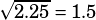A square and a regular hexagon have the same perimeter. If the area of the square is 2.25, what is the area of the hexagon?

A) 2.250
B) 2.598
C) 2.838
D) 3.464
E) 3.375

The sides of the square are, so the perimeter of the square is 4(1.5) = 6.

So the hexagon, which also has perimeter 6, will have sides of length 1.

To find the area of a regular hexagon, break it into 6 equilateral triangles:Each of those can further be broken into 30º-60º-90º triangles, because all the angles in an equilateral triangle are 60º.It’s easy enough to calculate the area of each of those: